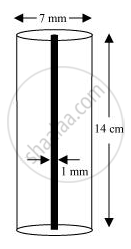# A lead pencil consists of a cylinder of wood with solid cylinder of graphite filled in the interior. The diameter of the pencil is 7 mm and the diameter of the graphite is 1 mm. If the length of the pencil is 14 cm, find the volume of the wood and that of the graphite. - Mathematics

A lead pencil consists of a cylinder of wood with solid cylinder of graphite filled in the interior. The diameter of the pencil is 7 mm and the diameter of the graphite is 1 mm. If the length of the pencil is 14 cm, find the volume of the wood and that of the graphite.

["Assume "pi=22/7]

#### SolutionRadius (r1) of pencil = (7/2)mm = (0.7/2)cm = 0.35 cm

Radius (r2) of graphite = (1/2)mm = (0.1/2)cm = 0.05 cm

Height (h) of pencil = 14 cm

"Volume of wood in pencil "=pi(r_1^2-r_2^2)h

=[22/7{(0.35)^2-(0.05)^2xx14}]cm^3

=[22/7(0.1225-0.0025)xx14]cm^3

= (44 x 0.12) cm3

= 5.28 cm3

"Volume of graphite "=pir_2^2h=[22/7xx(0.05)^2xx14]cm^3

= (44 x 0.0025) cm3

= 0.11 cm3

Concept: Volume of a Cylinder
Is there an error in this question or solution?
Chapter 13: Surface Area and Volumes - Exercise 13.6 [Page 231]

#### APPEARS IN

NCERT Class 9 Maths
Chapter 13 Surface Area and Volumes
Exercise 13.6 | Q 7 | Page 231

Share